﻿ Combining String Functions
Liquid Studio Documentation
Data Mapping / Combining String Functions
In This Topic
Combining String Functions
In This Topic

Using individual functions is often self-explanatory, but the Data Mapper is most useful when you combine functions. Here we are going to combine some functions to achieve a typical String transformation. For example, the Upper and Lower functions naturally convert input Strings to upper and lower case. However, you may find yourself in a position where you have to convert certain characters but not others. In such cases you can make use of multiple functions to achieve the desired result.

Let's assume that we want to map a text String so that the initial character is in upper case, but only the initial character. All other characters must be mapped in lower case. We will use school_source.xsd, a schema inferred from the following input XML for demonstration, in which the text includes a mix of upper and lower case characters:

Source XML
Copy Code
```<school>
<classroom door_number="14">
<pupil>
<name>arTHur</name>
<age>12</age>
</pupil>
<pupil>
<name>tOby</name>
<age>11</age>
</pupil>
<pupil>
<name>joNatHON</name>
<age>12</age>
</pupil>
</classroom>
<classroom door_number="21">
<pupil>
<name>maRY</name>
<age>12</age>
</pupil>
<pupil>
<name>jiLL</name>
<age>11</age>
</pupil>
</classroom>
</school>
```

We're intentionally including lower case initial letters with arbitrary upper case letters to demonstrate the function we are building here. The output XML will have the following structure, defined in school_target.xsd:

Target XML
Copy Code
```<institution>
<class>
<room>364</room>
<child c_age="14"><c_name>Thomas</c_name></child>
<child c_age="15"><c_name>Michael</c_name></child>
<child c_age="14"><c_name>Sarah</c_name></child>
</class>
<class>
<room>180</room>
<child c_age="14"><c_name>Jennifer</c_name></child>
<child c_age="15"><c_name>Claire</c_name></child>
</class>
</institution>
```

First, drag your source and target onto the Mapper area, selecting sample data and inferring Schema definitions for each if necessary. Match up all items except the "pupil/child" names: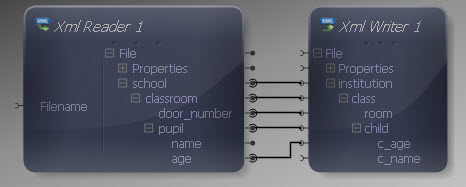Everything except the first character is going to be mapped in lower case, so let's convert the whole String to lower case to begin with. Drag the Lower function into the Mapper from the String section of the Component Palette.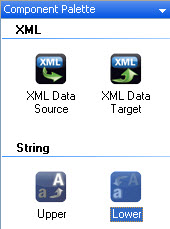Connect the Lower input to the "name" output in the XML Reader. This gives us a reference to the entire String in lower case. Later we will concatenate the first character, converted to upper case, with the rest of the String, left in lower case.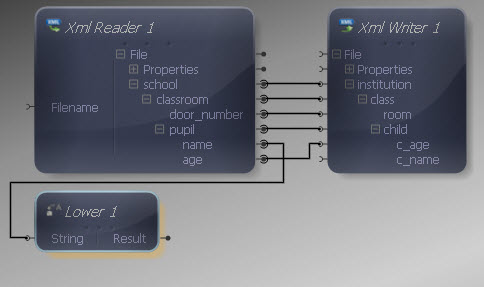Let's handle converting the first character to upper case. Add a Left function to your Mapper, connecting the output of Lower to its String input.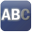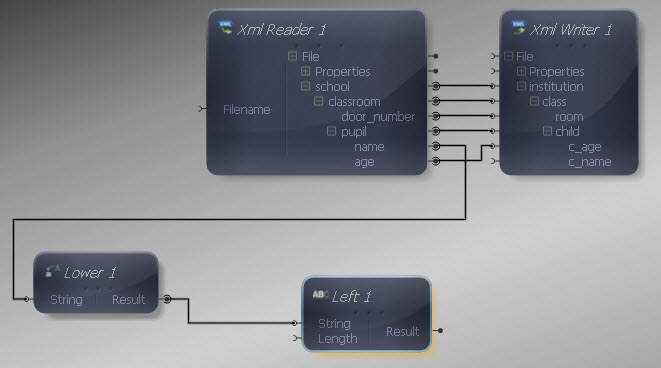We only want the first character, so the length is going to be 1. To specify this, drag a Constant Value from the Data Type section. Right-click the Constant and select Show Properties. Choose one of the integer data types and enter "1" as the value.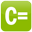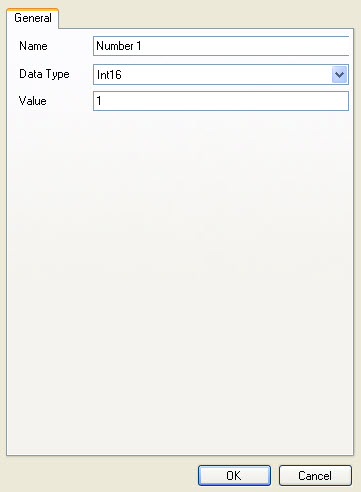Connect the Constant output to the Left Length input.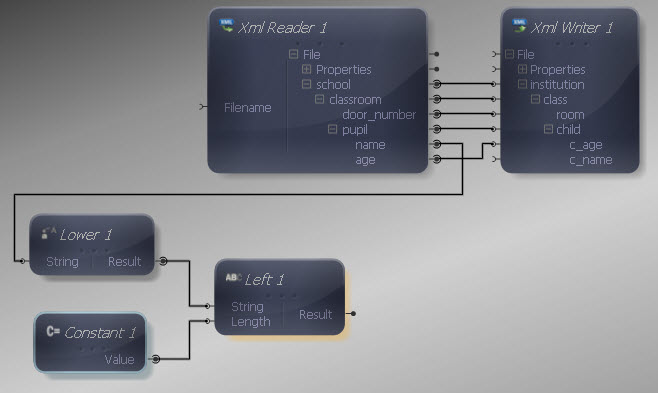Now we have the first character, let's convert it to upper case by dragging an Upper function over and connecting its input to the Left output.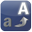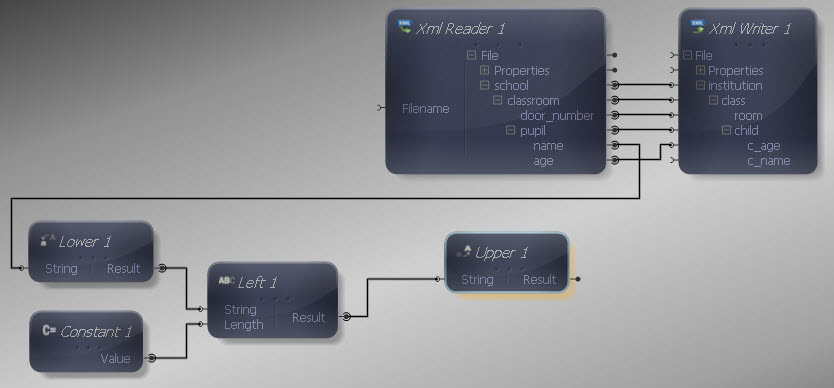Now we need to combine our upper case initial letter with the rest of the String before we can feed it into the XML Writer. To do this, drag Concat onto the Mapper and connect the Upper output to its first String input.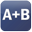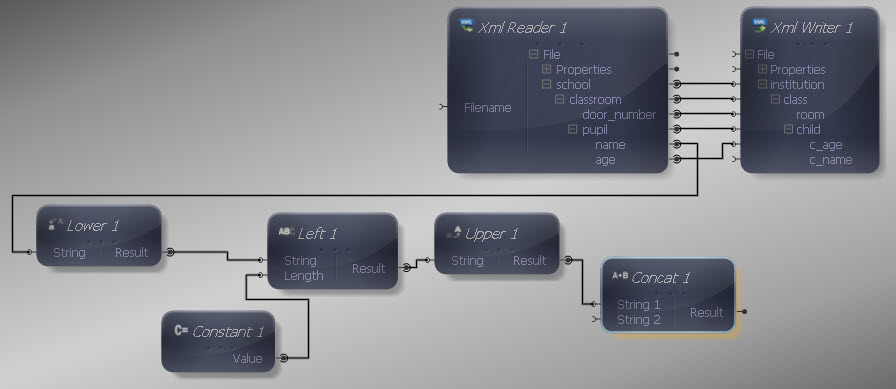Now we need to retrieve the second part of the input String, i.e. the part after the first character. Let's use a Right function, connecting its output to the second String Concat input.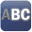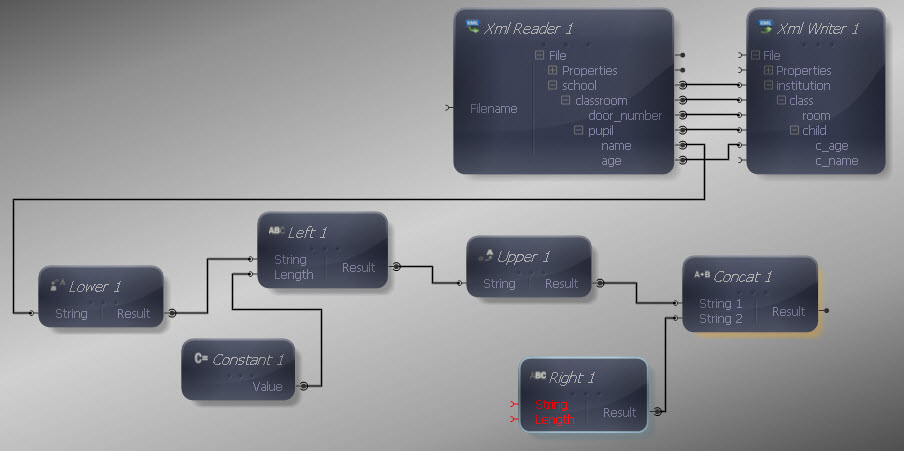Connect the Right String input to the output from the Lower function.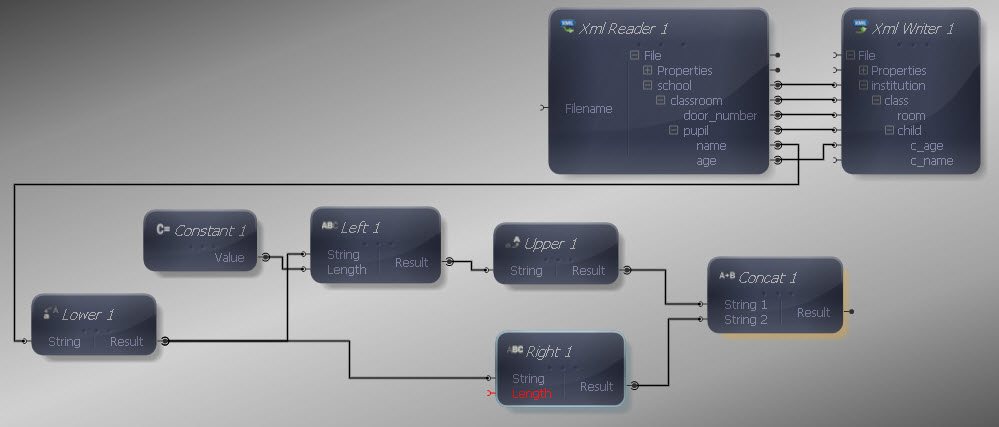Now we need to specify a length representing the original String minus one, so that we ignore the initial letter, which we are converting to upper case and feeding into the Concat function already. First get the length of the original String by dragging a Length function over and connecting its input to the Lower output.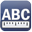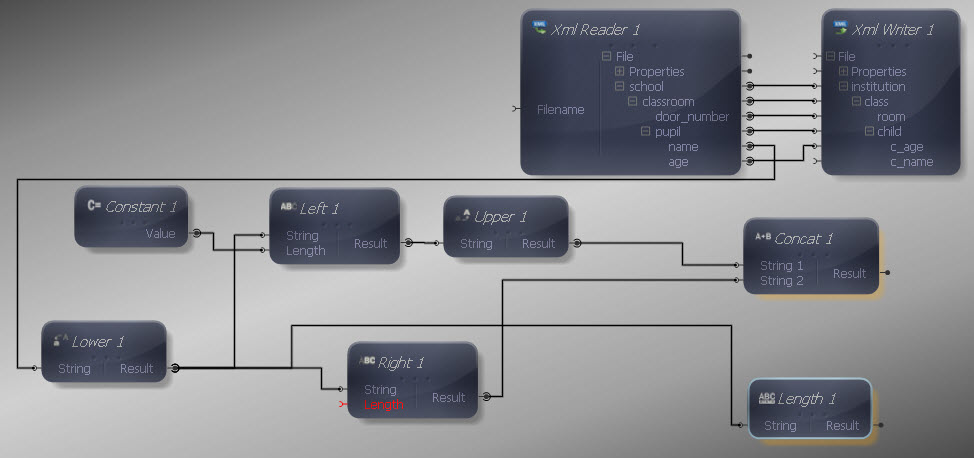Now we need to subtract 1 from the length in order to feed the correct number into the Right function. Drag a Subtract function from the Maths section. Connect the Length output to the first Subtract input. We already have a Constant Value for the number 1, so let's connect its output to the second Subtract input.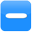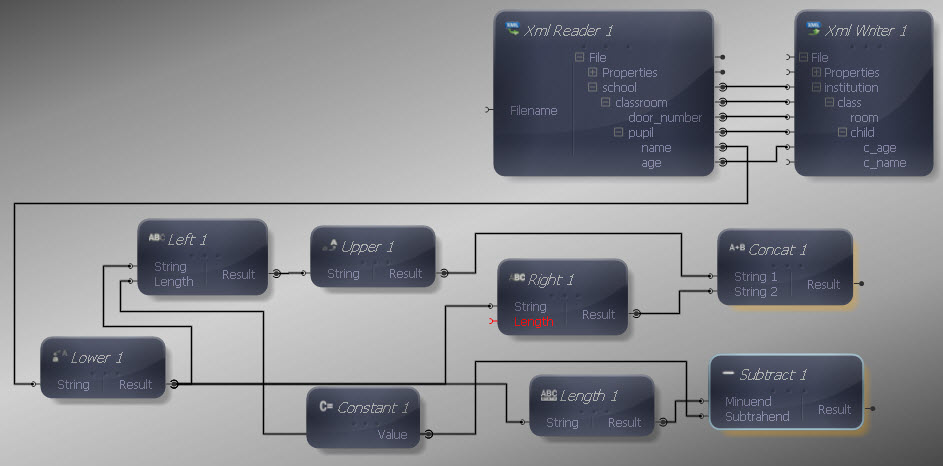Now the output of the Subtract function is the value we want to use as the length for the second part of the String. The result of the Right function is what we want the second part of our concatenation operation to use, so connect the Subtract output to the Length input of the Right function.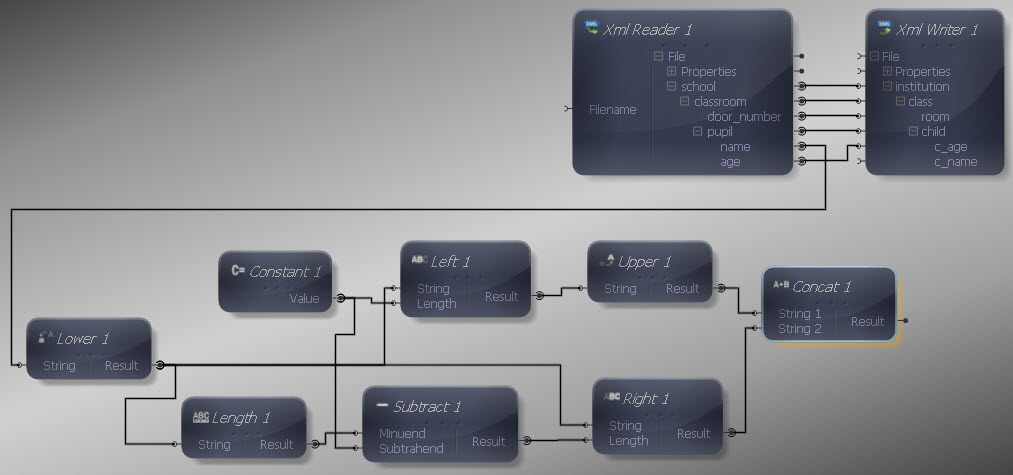Finally, connect the Concat output to the XML Writer input to complete the mapping definition.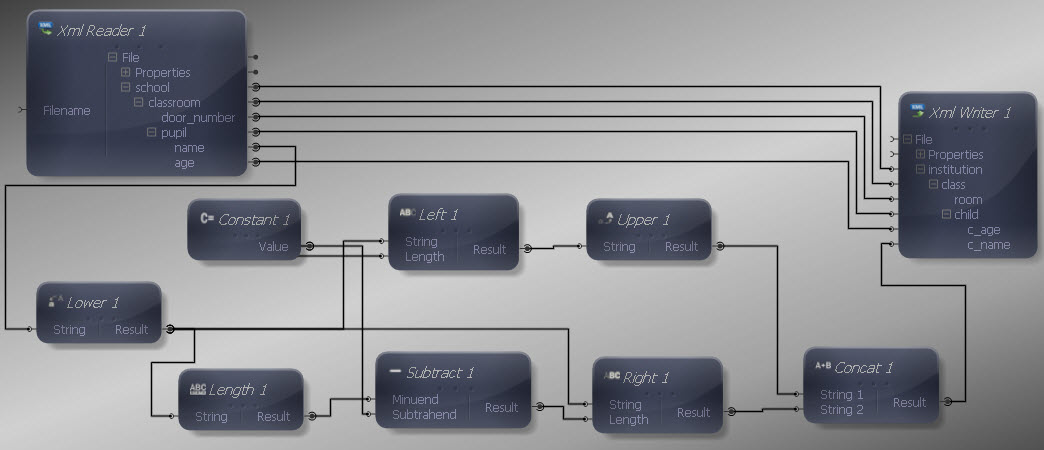Execute the mapping (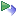) and you should receive the following output:

Output XML
Copy Code
```<institution>
<class>
<room>14</room>
<child c_age="12">
<c_name>Arthur</c_name>
</child>
<child c_age="11">
<c_name>Toby</c_name>
</child>
<child c_age="12">
<c_name>Jonathon</c_name>
</child>
</class>
<class>
<room>21</room>
<child c_age="12">
<c_name>Mary</c_name>
</child>
<child c_age="11">
<c_name>Jill</c_name>
</child>
</class>
</institution>
```

In the above example we could have alternatively used the SubString function instead of the Left and Right functions. With SubString, the inputs are the String, the start position and end position. This means that in the above case we would have still needed the Length function, and could have used the number 1 Constant together with an additional zero Constant to specify the start and end indices for the two parts of the String.

If you think of your data mapping actions in terms of what their inputs and outputs are in each case, you will develop and intuitive sense of how to use the functions effectively. Other String functions to try combining include Replace (which replaces every instance of a Search String in the target String), the Trim functions (which get rid of whitespace, essential when transferring data from A to B) and the CodeToChar/ CharToCode functions (which transform values between character and Unicode data).

XML data comes from many different types of source and is used in a host of application contexts, so being able to transform your text Strings in a variety of ways is often vital.

The string manipulation performed in the sample above results in a fairly complex transform. In order to simplify this is possible to convert it into one or more Sub Functions, which can then be re-used in other transforms.